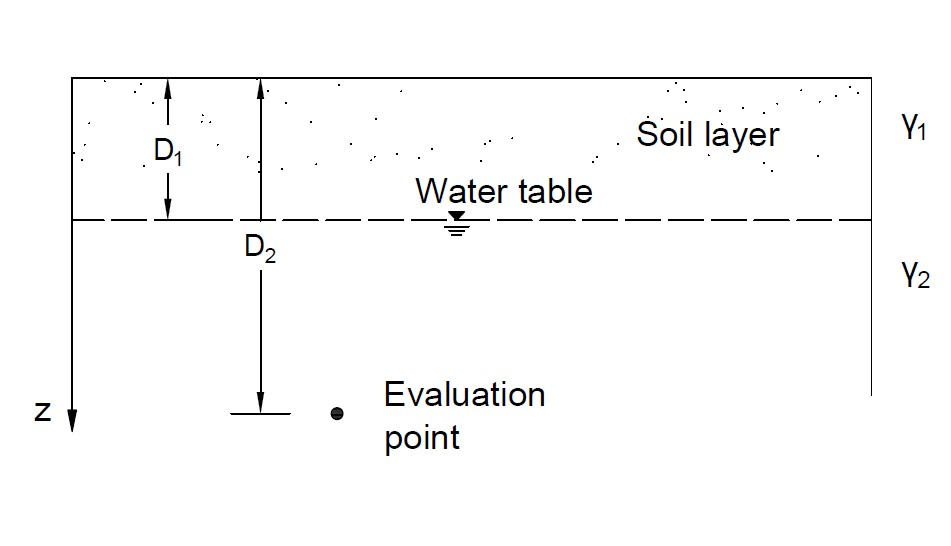# Dilatometer Test Tool## Geometry of Dilatometer Test## Tips

• This tool is for obtaining mechanical properties of soils through the dilatometer test, as shown in the figure above.
• All the input parameters should be positive.
• The unit weight of water is 9.8 kN/m3.
• The calculation can be used for sandy layer or clayey soil.
• If no water is concerned, the underground water table can be set lower than the evaluation point.
• The calculation is mainly based on the correlations proposed by Marchetti(1980). The undrained cohesions obtained under diffferent coditions are different.
• For "nan", "0" or "inf" displayed in Results, please check your input parameters.

D1 (m)
D2 (m)
γ1 (kN/m3)
γ2 (kN/m3)
p0 (kN/m2)
p1 (kN/m2)
μs

## Output Results

• For all soils
Coefficient of at rest earth pressure
K0 =
Modulus of elasticity
Es (kN/m2) =
• For clayey soil
Overconsolidation ratio
OCR =
cu (Marchetti normally consolidated)
cu (kN/m2) =
cu (Marchetti overconsolidated)
cu (kN/m2) =
cu (Kamei Method)
cu (kN/m2) =
• For sandy soil
Friction angle
φ (°) =